# RD Sharma Class 10 Ex 9.1 Solutions Chapter 9 Arithmetic Progressions

In this chapter, we provide RD Sharma Class 10 Ex 9.1 Solutions Chapter 9 Arithmetic Progressions for English medium students, Which will very helpful for every student in their exams. Students can download the latest RD Sharma Class 10 Ex 9.1 Solutions Chapter 9 Arithmetic Progressions pdf, Now you will get step by step solution to each question.

# Chapter 9: Arithmetic Progressions Exercise – 9.1

### Question: 1

Write the first terms of each of the following sequences whose nth term are

(i) an = 3n + 2

(iii) an = 3n

(v) an = (-1)n2n

(vii) an = n2 – n + 1

(viii) an = n2 – n + 1

### Solution:

We have to write first five terms of given sequences

(i) an = 3n + 2

Given sequence an = 3n + 2

To write first five terms of given sequence put n = 1, 2, 3, 4, 5, we get

a1 = (3 × 1) + 2 = 3 + 2 = 5

a2 = (3 × 2) + 2 = 6 + 2 = 8

a3 = (3 × 3) + 2 = 9 + 2 = 11

a4 = (3 × 4) + 2 = 12 + 2 = 14

a5 = (3 × 5) + 2 = 15 + 2 = 17

∴ The required first five terms of given sequence an = 3n + 2 are 5, 8, 11, 14, 17.

Given sequence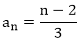Put n = 1, 2, 3, 4, 5 then we get

∴ The required first five terms of given sequence

(iii) an = 3n

Given sequence an = 3n

To write first five terms of given sequence, put n = 1, 2, 3, 4, 5 in given sequence then,

a1 = 31 = 3; a2 = 32 = 9; a3 = 27; a4 = 34 = 81; a5 = 35 = 243.

Given sequence,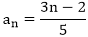To write first five terms, put n = 1, 2, 3, 4, 5 in given sequenceThen, we get

∴ The required first five terms are 1/5, 4/5, 7/5, 10/5, 13/5

(v) an = (-1)n2n

Given sequence is an = (-1)n2n

To get first five terms of given sequence an, put n = 1, 2, 3, 4, 5.

a1 = (-1)1.21 = (-1).2 = -2

a2 = (-1)2.22 = (-1).4 = -4

a3 = (-1)3.23 = (-1).8 = -8

a4 = (-1)4.24 = (-1).16 = 16

a5 = (-1)5.25 = (-1).32 = -32

∴ The first five terms are – 2, 4, – 8, 16, – 32.

The given sequence is,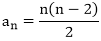To write first five terms of given sequencePut n = 1, 2, 3, 4, 5. Then, we get

∴  The required first five terms are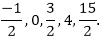(vii) an = n2 – n + 1

The given sequence is, an = n2 – n + 1

To write first five terms of given sequence an1 we get put n = 1, 2, 3, 4, 5. Then we get

a1 = 12 – 1 + 1 = 1

a2 = 22 – 2 + 1 = 3

a3 = 32 – 3 + 1 = 7

a4 = 42 – 4 + 1 = 13

a5 = 52 – 5 + 1 = 21

∴ The required first five terms of given sequence an = n2 – n + 1 are 1, 3, 7, 13, 21

(viii) an = 2n2 – 3n + 1

The given sequence is an = 2n2 – 3n + 1

To write first five terms of given sequence and, we put n = 1, 2, 3, 4, 5. Then we get

a1 = 2.12 – 3.1 + 1 = 2 – 3 + 1 = 0

a2 = 2.22 – 3.2 + 1 = 8 – 6 + 1 = 3

a3 = 2.32 – 3.3 + 1 = 18 – 9 + 1 = 10

a4 = 2.42 – 3.4 + 1 = 32 – 12 + 1 = 21

a5 = 2.52 – 3.5 + 1 = 50 – 15 + 1 = 36

∴ The required first five terms of given sequence an – 2n2 – 3n + 1 are 0, 3, 10, 21, 36

Given sequence is,To write first five terms of given sequence we put n = 1, 2, 3, 4, 5. Then, we get,

∴ The required first five terms of given sequence

### Question: 2

Find the indicated terms in each of the following sequences whose nth terms are:

(i) an = 5n – 4; a12 and a15

(iii) an = n(n – 1)(n – 2); a5 and a8

(iv) an = (n – 1)(2 – n)(3 + n);a11 a21 a3

(v) an = (-1)nn;a3, a5, a8

### Solution:

We have to find the required term of a sequence when nth term of that sequence is given:

(i) an = 5n – 4; a12 and a15

Given nth term of a sequence an = 5n – 4

To find 12th terms, 15th terms of that sequence, we put n = 12 15 in its nth term.

Then, we get

a12 = 5.12 – 4 = 60 – 4 = 56

a15 = 5.15 – 4 = 15 – 4 = 71

∴ The required terms a12 = 56, a15 = 71

Given nth term is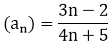To find 7th, 8th terms of given sequence, we put n = 7, 8.

∴ The required terms

(iii) an = n(n – 1)(n – 2); a5 and a8

Given nth term is an = n(n – 1)(n – 2)

To find 5th, 8th terms of given sequence, put n = 5, 8 in an then, we get

a5 = 5(5 – 1).(5 – 2) = 5.4.3 = 60

a8 = 8.(8 – 1).(8 – 2) = (8.7.6) = 336

∴ The required terms are a5 = 60 and a8 = 336

(iv) an = (n – 1)(2 – n)(3 + n);a11 a21 a3

The given nth term is an = (n + 1)(2 – n)(3 + n)

To find a1, a2, a3 of given sequence put n = 1, 2, 3 is an

a1 = (1 – 1)(2 – 1)(3 + 1) = 0.1.4 = 0

a2 = (2 – 1)(2 – 2)(3 + 2) = 1.0.5 = 0

a3 = (3 – 1)(2 – 3)(3 + 3) = 2.-1.6 = -12

∴ The required terms a1 = 0, a2 = 0, a3 = -12

(v) an = (-1)nn; a3, a5, a8

The given nth term is, an = (-1)n.n

To find a3, a5, a8 of given sequence put n = 3, 5, 8, in an.

a3 = (-1)3.3 = -1.3 = -3

a5 = (-1)5.5 = -1.5 = -5

a8 = (-1)8 = 1.8 = 8

∴ The required terms a3 = -3, a5 = -5, a8 = 8

### Question: 3

Find the next five terms of each of the following sequence given by:

(i) a1 = 1, 1n = an-1 + 2, n ≥ 2

(ii) a1 = a2 = 2, an = an-1 – 3, n > 2

(iv) a1 = 4, an = 4 an-1 + 3, n > 1

### Solution:

We have to find next five terms of following sequences.

(i) a1 = 1, an = an-1 + 2, n ≥ 3

Given first term (a1) = 1,

nth term an = an-1 + 2, n ≥ 2

To find 2nd, 3rd, 4th, 5th, 6th terms, we use given condition n ≥ 2 for nth term an = an-1 + 2

a2 = a2-1 + 2 = a1 + 2 = 1 + 2 = 3 (∴ a1 = 1)

a3 = a3-1 + 2 = a2 + 2 = 3 + 2 = 5

a4 = a4-1 + 2 = a3 + 2 = 5 + 2 = 7

a5 = a5-1 + 2 = a4 + 2 = 7 + 2 = 9

a6 = a6-1 + 2 = a5 + 2 = a + 2 = 11

∴ The next five terms are,

a2 = 3, a3 = 5, a4 = 7, a5 = a, a6 = 11

(ii) a1 = a2 = 2, an = an-1 – 3, n > 2

Given,

First term (a1) = 2

Second term (a2) = 2

nth term (an) = an-1 – 3

To find next five terms i.e., a3, a4, a5, a6, a7 we put n = 3, 4, 5, 6, 7 is an

a3 = a3-1 – 3 = 2 – 3 = – 1

a4 = a4-1 – 3 = a3 – 3 = – 1 – 3 = – 4

a5 = a5-1 – 3 = a4 – 3 = -4 – 3 = -7

a6 = a6-1 – 3 = a5 – 3 = -7 – 3 = -10

a7 = a7-1 – 3 = a6 – 3 = -10 – 3 = -13

∴ The next five terms are, a3 = -1, a4 = – 4, a5 = -7, a6 = -10, a7 = -13

Given, first term (a1) = – 1

To find next five terms i.e., a2, a3, a4, a5, a6 we put n = 2, 3, 4, 5, 6 is an

∴ The next five terms are,

(iv) a1 = 4, an = 4 an-1 + 3, n > 1

Given,

First term (a1) = 4

nth term (an) = 4 an-1 + 3, n > 1

To find next five terms i.e., a2, a3, a4, a5, a6 we put n = 2, 3, 4, 5, 6 is an

Then, we get

a2 = 4a2-1 + 3 = 4. a1 + 3 = 4.4 + 3 = 19 (∴ a1 = 4)

a3 = 4a3-1 + 3 = 4.a2 + 3 = 4(19) + 3 = 79

a4 = 4a4-1 + 3 = 4.a3 + 3 = 4(79) + 3 = 319

a5 = 4a5-1 + 3 = 4.a4 + 3 = 4(319) + 3 = 1279

a6 = 4a6-1 + 3 = 4.a5 + 3 = 4(1279) + 3 = 5119

∴ The required next five terms are,

a2 = 19, a3 = 79, a4 = 319, a5 = 1279, a6 = 5119

All Chapter RD Sharma Solutions For Class10 Maths

I think you got complete solutions for this chapter. If You have any queries regarding this chapter, please comment on the below section our subject teacher will answer you. We tried our best to give complete solutions so you got good